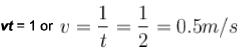Courses

# Waves NAT Level - 1

## 10 Questions MCQ Test Topic wise Tests for IIT JAM Physics | Waves NAT Level - 1

Description
This mock test of Waves NAT Level - 1 for Physics helps you for every Physics entrance exam. This contains 10 Multiple Choice Questions for Physics Waves NAT Level - 1 (mcq) to study with solutions a complete question bank. The solved questions answers in this Waves NAT Level - 1 quiz give you a good mix of easy questions and tough questions. Physics students definitely take this Waves NAT Level - 1 exercise for a better result in the exam. You can find other Waves NAT Level - 1 extra questions, long questions & short questions for Physics on EduRev as well by searching above.
*Answer can only contain numeric values
QUESTION: 1

### Plane harmonic waves of frequency 500 Hz are produced in air with displacement amplitude 1 x 10-3 cm. Deduce energy flux in J/m2) in the wave (density of air =1.29 gm/lit, speed of sound in air = 340 m/s).

Solution: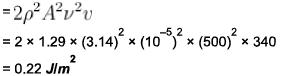*Answer can only contain numeric values
QUESTION: 2

### Plane harmonic waves of frequency 500 Hz are produced in air with displacement amplitude 1 x 10-3 cm.  Deduce pressure amplitude (in N/m2).  (density of air = 1.29 gm/lit, speed of sound in air = 340m/s)

Solution:

Given A = 1 x 10-3 cm = 10-5 m, v = 500 Hz, v = 340 m/s, p = 1.29 g/lit = 1.29 kg/m3
Pressure amplitude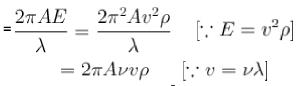= 2 x 3.14 x 10-5 x 500 x 340 x 1.29
= 13.8 N/m2

*Answer can only contain numeric values
QUESTION: 3

### If the frequency of a tuning fork is 400 Hz and the velocity of sound in air is 320 m/s, find how far does the sound travel (in m) while the fork completes 30 vibrations?

Solution: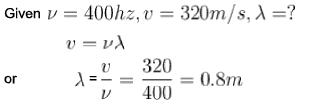∴ Distance travelled by the wave when the fork completes 1 vibration = 0.8 m.
So distance travelled by the wave when the fork completes 30 vibrations.
= 0.8 x 30
= 24m

*Answer can only contain numeric values
QUESTION: 4

The vibrations of a string of length 60 cm fixed at both ends are represented by the equation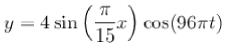where x and y are in cm and t in seconds. What is the maximum displacement of point x = 5cm ?

Solution:

The given equation is :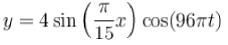This can be written as :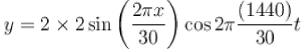This shows that a = 2 cm, λ =30 cm and ω = 1440 cm/s
The maximum displacement is given by :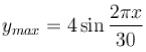For x = 5 cm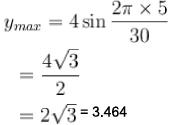*Answer can only contain numeric values
QUESTION: 5

A transverse harmonic wave of amplitude 0.02 m is generated at one end (x = 0) along horizontal string by a tuning fork of frequency 500 Hz. At a given instant of time, the displacement of the particle at x = 0.1 m is 0.005 m and that of the particle at x = 0.2 m is 0.005 m. Calculate the wavelength of the wave?

Solution:

The general equation of this wave is given by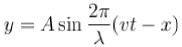Here A = 0.02 m
Again, when x = 0.1m, y = -0.005 m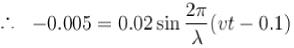or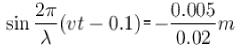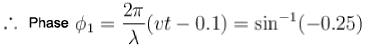Again x = 0.2m, y = 0.005m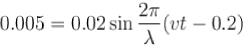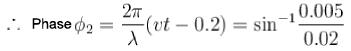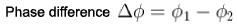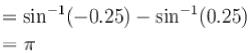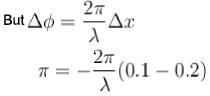or λ = 0.2m

*Answer can only contain numeric values
QUESTION: 6

Equations of a stationary and a travelling waves are as follows :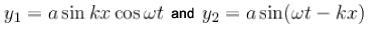The phase difference between two points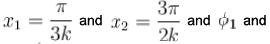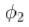respectively for the two waves. The ratio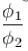is :

Solution: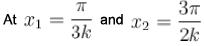sin kx1, or sin kx2 is not zero.
Therefore, neither of x1 or x2 is a node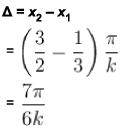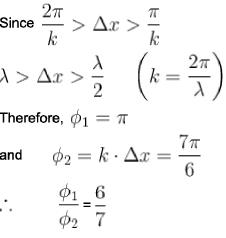*Answer can only contain numeric values
QUESTION: 7

A simple harmonic wave travelling x-axis is given by y = 5 sin 2π ( 0.2t - 0.5x) [x is in m and t in s]. Calculate the amplitude (in m ).

Solution:

Here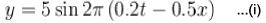But the standard progressive wave equation is :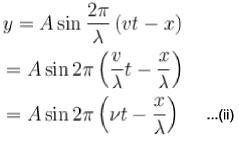Comparing equation (i) and (ii), we have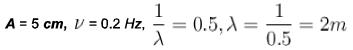*Answer can only contain numeric values
QUESTION: 8

The equation for the vibration of a string fixed at both ends vibrating in its third harmonic is given by :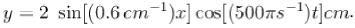The length of the string is :

Solution: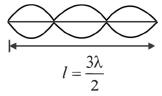Wave number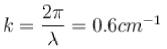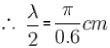or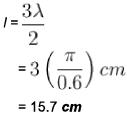*Answer can only contain numeric values
QUESTION: 9

A wave of frequency 400 Hz is travelling with a velocity 800 m/s. How far are two points situated whose displacement differs in phase by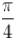?

Solution: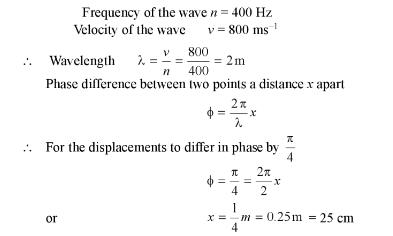*Answer can only contain numeric values
QUESTION: 10

The amplitude of wave disturbance propagating in positive x-axis is given by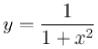at t = 0 and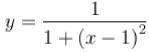at t= 2s, where x and y are in meters. The shape of the disturbance does not change during the propagation. The velocity (in m/s) of the wave is :

Solution:

The given pulse is of the form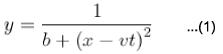where v is the wave velocity
Given equation is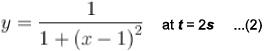Comparing eq. (1) and (2), we get